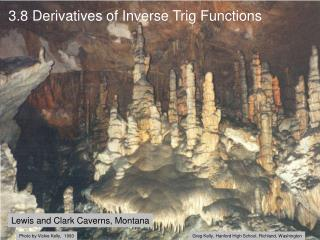# 3.8 Derivatives of Inverse Trig Functions - PowerPoint PPT PresentationDownload Presentation3.8 Derivatives of Inverse Trig Functions

Presentation Description
Download Presentation## 3.8 Derivatives of Inverse Trig Functions

- - - - - - - - - - - - - - - - - - - - - - - - - - - E N D - - - - - - - - - - - - - - - - - - - - - - - - - - -
##### Presentation Transcript

1. Photo by Vickie Kelly, 1993 Greg Kelly, Hanford High School, Richland, Washington 3.8 Derivatives of Inverse Trig Functions Lewis and Clark Caverns, Montana

2. At x = 2: We can find the inverse function as follows: To find the derivative of the inverse function: Switch x and y.

3. Slopes are reciprocals. At x = 2: At x = 4:

4. Slopes are reciprocals. The derivative of Derivative Formula for Inverses: evaluated at the derivative of evaluated at . Because x and y are reversed to find the reciprocal function, the following pattern always holds: is equal to the reciprocal of

5. Given: Find: Derivative Formula for Inverses: A typical problem using this formula might look like this:

6. We can use implicit differentiation to find:

7. But so is positive. We can use implicit differentiation to find:

8. We could use the same technique to find and . d - 1 sec x dx

9. Your calculator contains all six inverse trig functions. However it is occasionally still useful to know the following: p